featuresarchitecture

# Understanding Subgraph in NebulaGraph 2.0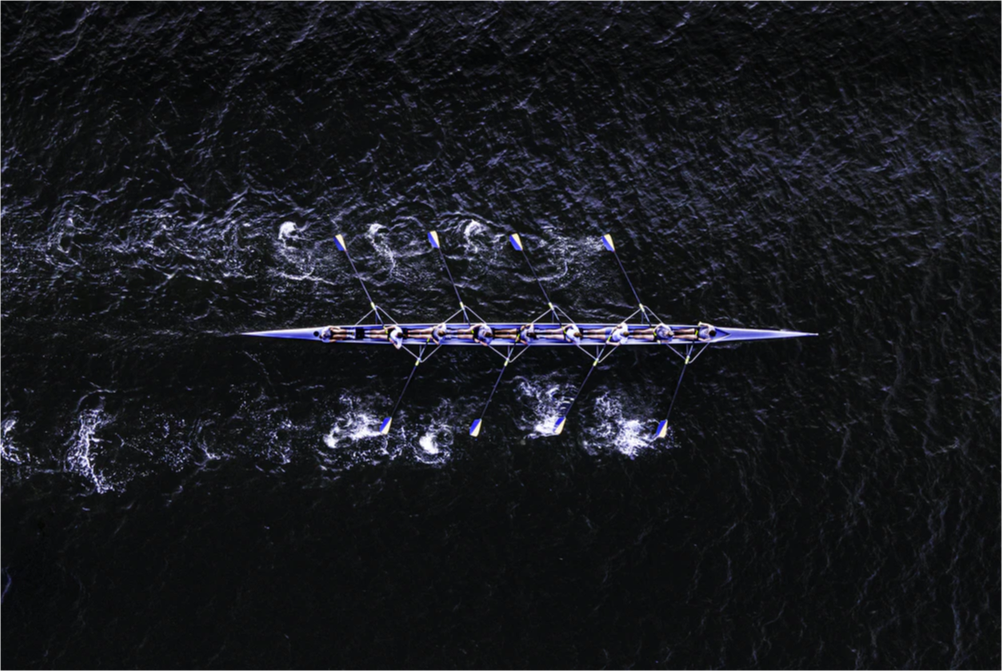## Introduction

In An Introduction to NebulaGraph 2.0 Query Engine, I introduced how the query engine differs between V2.0 and V1.0 of NebulaGraph.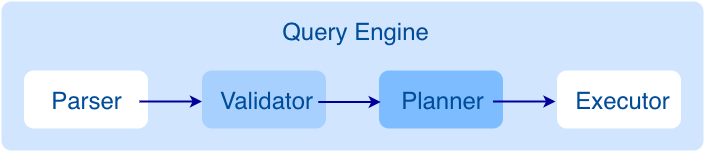As shown in the preceding figure, you can see that when a query statement is sent from the client, how the query engine parses the statement, generates an AST, validates the AST, and then generates an execution plan. In this article, I will introduce more about the query engine through the new subgraph feature in NebulaGraph 2.0 and focus on the execution plan generation process, to help you understand the source code better.

## Definition of Subgraph

A subgraph of a graph is composed of a subset of the graph's vertices and a subset of its edges. When a starting vertex is given, a traversal starts from it and expands following the specified edge type until all the vertices at the specified number of hops are reached. All the reachable vertices and edges form a subgraph.

## Syntax of Subgraph

``````GET SUBGRAPH [<step_count> STEPS] FROM {<vid>, <vid>...} [IN <edge_type>, <edge_type>...]
[OUT <edge_type>, <edge_type>...] [BOTH <edge_type>, <edge_type>...]
``````
• step_count: Specifies the number of hops from the starting vertex to obtain a subgraph consisting of vertices and edges that are reached at 0 to `step_count` hops. It must be a non-negative integer. The default value is 1.
• vid: Specifies the ID of the starting vertex.
• edge_type: Specifies the edge type. `IN`, `OUT`, and `BOTH` can be added to specify the direction of the edges from the starting vertex. By default, `BOTH` is used.

## Implementation of Subgraph

When the query engine receives a `GET SUBGRAPH` statement, the Parser, composed of Flex and Bison, will extract the necessary content from the query statement according to the specified rule, that is, the `get_subgraph_sentence` rule defined in parser.yy, and then generate an AST, as shown in the following figure.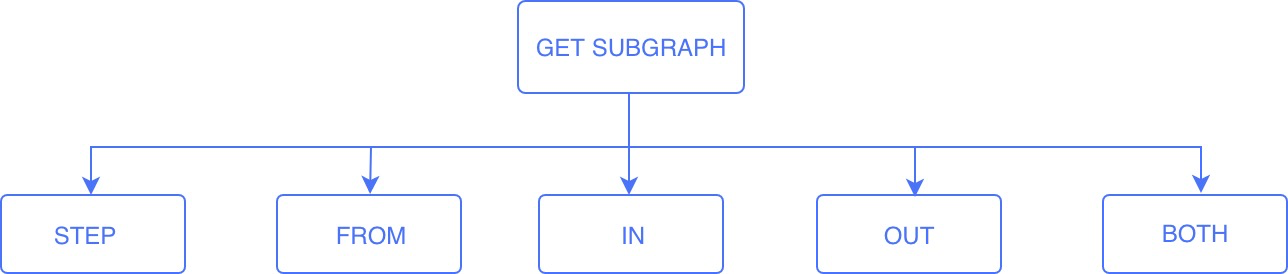And then in the Validate stage, the generated AST is validated, aiming to make sure that all the inputs from users conform to the syntax rule. For more information, see An Introduction to NebulaGraph 2.0 Query Engine. When the validation is successful, the content of the AST will be extracted and an execution plan will be generated.

How is the execution plan generated? Database solutions may differ in the form of the execution plan, but their implementations are the same, that is, depending on which operators are used and how the query layer interacts with the storage layer, because data needs to be taken from the storage layer for each query statement. In NebulaGraph, the query engine interacts with the storage layer through RPC (fbthrift), of which the interfaces are defined in the `interface` directory of the `common` repository. `getNeighbors` and `getProps` are the key interfaces.

In fbthrift, `getNeighbors` is defined as follows:

``````struct GetNeighborsRequest {
1: common.GraphSpaceID                      space_id,
2: list<binary>                             column_names,
3: map<common.PartitionID, list<common.Row>>
(cpp.template = "std::unordered_map")   parts,
4: TraverseSpec                             traverse_spec
}
``````

To understand the variables in the preceding structure, see the comments in the source code: https://github.com/vesoft-inc/nebula-common/blob/master/src/common/interface/storage.thrift.

It mainly aims to enable this process:

1. The query engine passes the starting vertex and the specified edge types according to the defined structure.
2. In the storage layer, the starting vertex is located.
3. The properties of the vertex and its outgoing edges are assembled into a table and the table is returned to the query engine. The table format is defined in `GetNeighborsResponse`. For more information, see https://github.com/vesoft-inc/nebula-common/blob/master/src/common/interface/storage.thrift.
4. The query engine extracts the necessary content from the table.

For example, for the NBA dataset, when `Tim Duncan` and `Manu Ginobili` are given as the starting vertices and `like` of both directions are given as the edge type, we can get the information of `\$^.player.name`, `like._dst`, `\$\$.player.name`, and `like.likeness` as follows: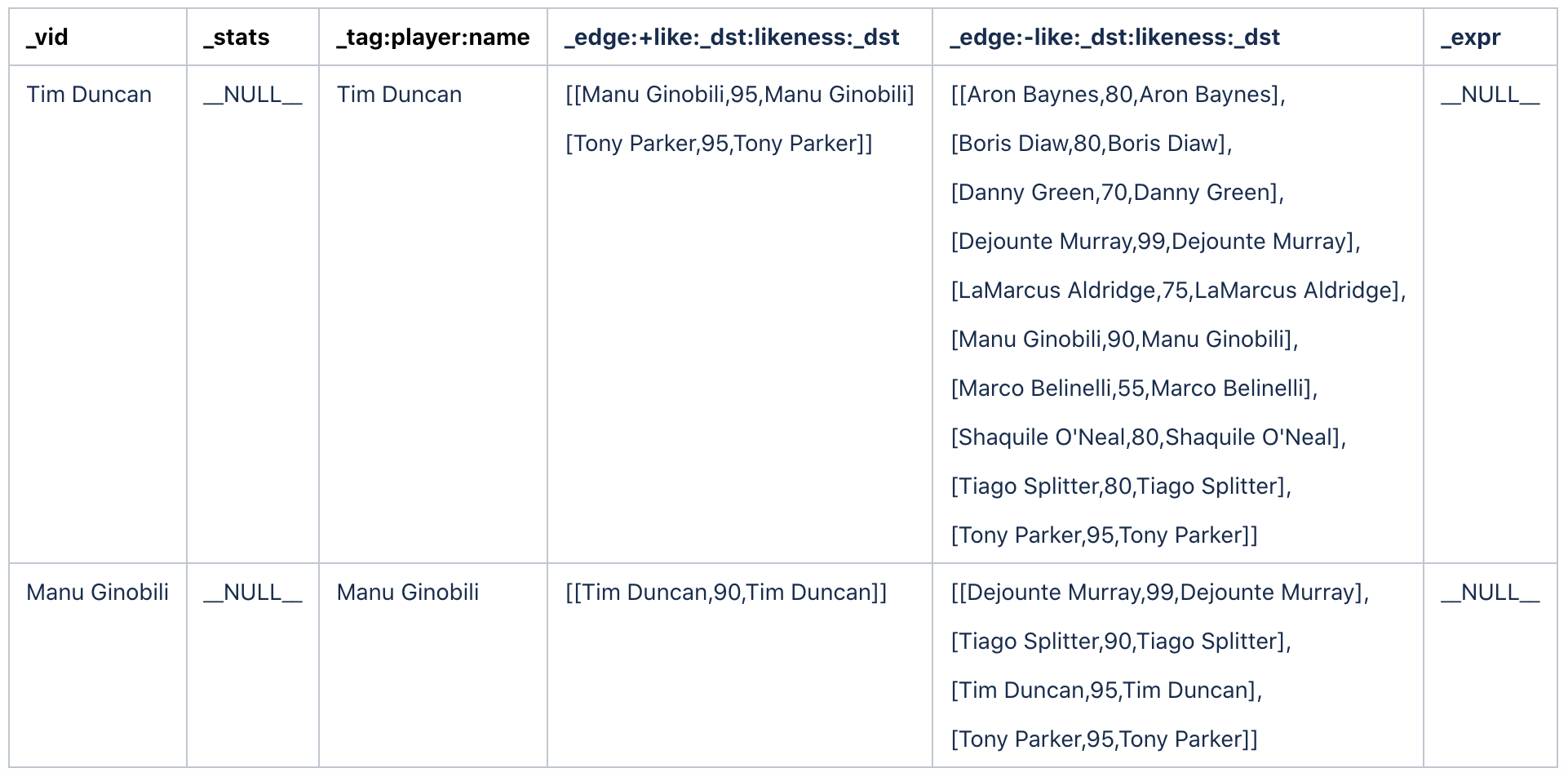Because the traversal is done in both directions, `+ like` in the fourth column of the preceding table represents outgoing edges, and `- like` in the fifth column represents incoming edges.

In NebulaGraph, edges are stored with their source vertices in the storage layer, so all the properties of a vertex and its outgoing edges can be obtained through the `GetNeighbor` interface. However, if you want to obtain the property information of the destination vertices of these edges, the `getProps` interface is needed. A `FETCH` statement also needs this interface to obtain the property information of a vertex or an edge. For more information about `GetPropRequest`, see https://github.com/vesoft-inc/nebula-common/blob/master/src/common/interface/storage.thrift.

### Execution Plan

With the preceding interfaces, an execution plan can be generated. The operators, `start`, `getNeighbor`, `subgraph`, `loop`, and `dataCollect`, are necessary.

• start: A leaf node of an execution plan. It does nothing but informs the scheduler that no more operator can be relied upon. In other words, this is the termination condition in the recursive algorithm.
• loop: Equivalent to the `WHILE` loop in C language. It is composed of three parts: `depend`, `condition`, and `loopBody`. `depend` is used in multi-statements and the PIPE and no more details about it is involved in this article. `condition` is equivalent to the termination condition. `loopBody` is equivalent to the loop body in a `WHILE` loop.
• subgraph: Responsible for extracting the properties of `_dst`, the destination vertex, from the `getNeighbor` result, filtering out the destination vertices that have been traversed to avoid repeatedly fetching data from the storage layer, and then using them as the input for the next hop executed by `getNeighbor`.
• datacollect: Responsible for assembling the properties of the reachable vertices and their edges into the vertices and edge types.

Let's take the graph in the following figure as an example to introduce how a subgraph is generated.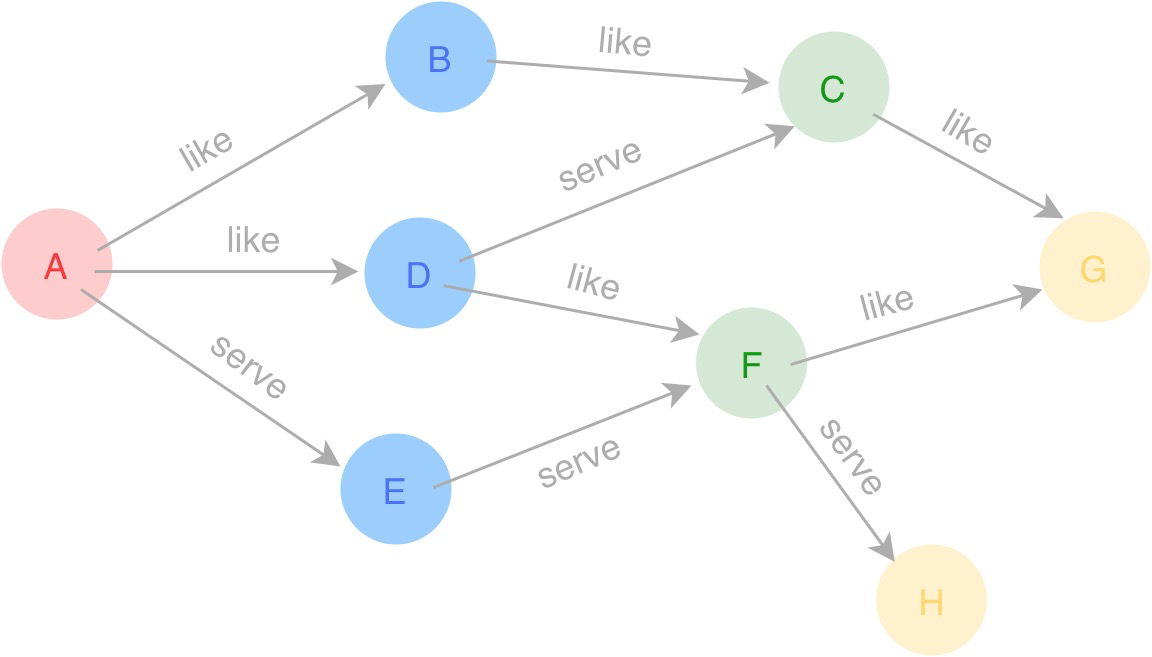Single-hop traversal

It is easy to start from Vertex A and traverse along the `like` edges at one hop for the vertices and edges. In this scenario, only getNeighbor and dataCollect are needed. The execution plan is as follows.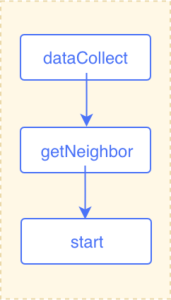Multi-hop traversal

The single-hop traversal is a special case of the multi-hop traversal, so it can be treated as part of a multi-hop traversal. In a three-hop traversal starting from Vertex A along the `like` edges at three hops, the destination vertices can be extracted after `getNeighbor` is executed and these vertices can be the starting vertices to call the `getNeighbor` interface. Two loops are sufficient for such a scenario. The number of hops can be set as the termination condition in `loop`. Therefore, the execution plan is as follows.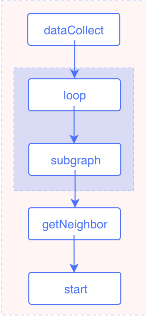Input and output

Generally, the input of each operator is the output of the one that it depends on, so according to the dependencies represented in the execution plan, you can see the input and output of each operator. However, in some cases, for example, in a multi-hop traversal, the input of each `getNeighbor` is the destination vertex that is reached following an edge, that is, the output of the `subgraph` operator, so the output of `subgraph` is the input of `getNeighbor`. Such a dependency is different from that shown in the preceding figure. Therefore, the input and output of each operator must be set separately. From An Introduction to NebulaGraph 2.0 Query Engine, you know that the input and output of each operator are stored in a hash table, of which values are of the `vector` type. The following ResultMap table is an example.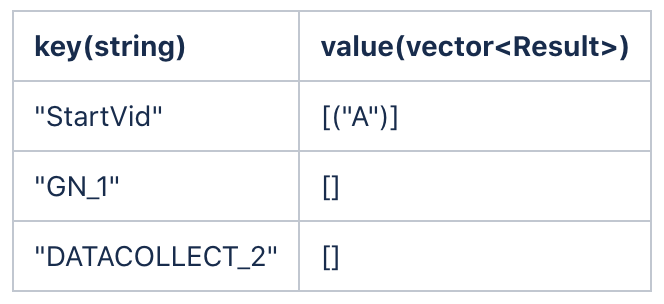• The starting vertex is stored in ResultMap["StartVid"].
• The input of getNeighbor is ResultMap["StartVid"] and its output is stored in ResultMap["GN_1"].
• The input of getNeighbor is ResultMap["StartVid"] and its output is stored in ResultMap["GN_1"].
• No data is generated by loop. It is used as a logic loop, so neither input nor output is necessary.
• The input of dataCollect is ResultMap["GN_1"] and its output is stored in ResultMap["DATACOLLECT_2"].

At this time, the getNeighbor operator puts the result of each loop at the end of the vector in ResultMap["GN_1"], and the subgraph operator takes the value from the end of the vector in ResultMap["GN_1"] and then stores the starting vertex for the next hop in ResultMap["StartVid"].

After the first hop, the result of ResultMap is as follows. ​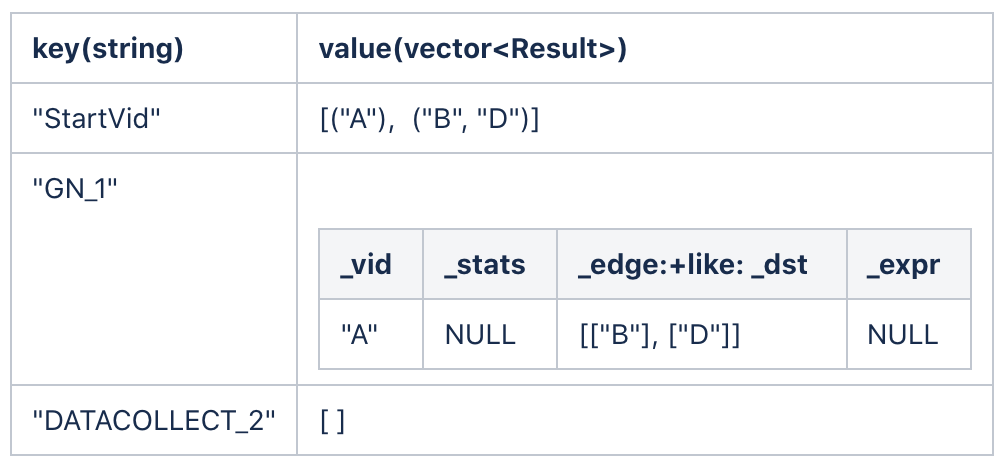To make the result clearer, only the properties of `_dst` are shown as the result of `getNeighbor`. In the actual result, the properties of all the edges and the starting vertex are listed, as show in Table 1.

`subgraph` receives "GN_1" as its input, extracts the properties of `_dst`, and then stores the result in "StartVid".

After the second hop traversal, the ResultMap is as follows.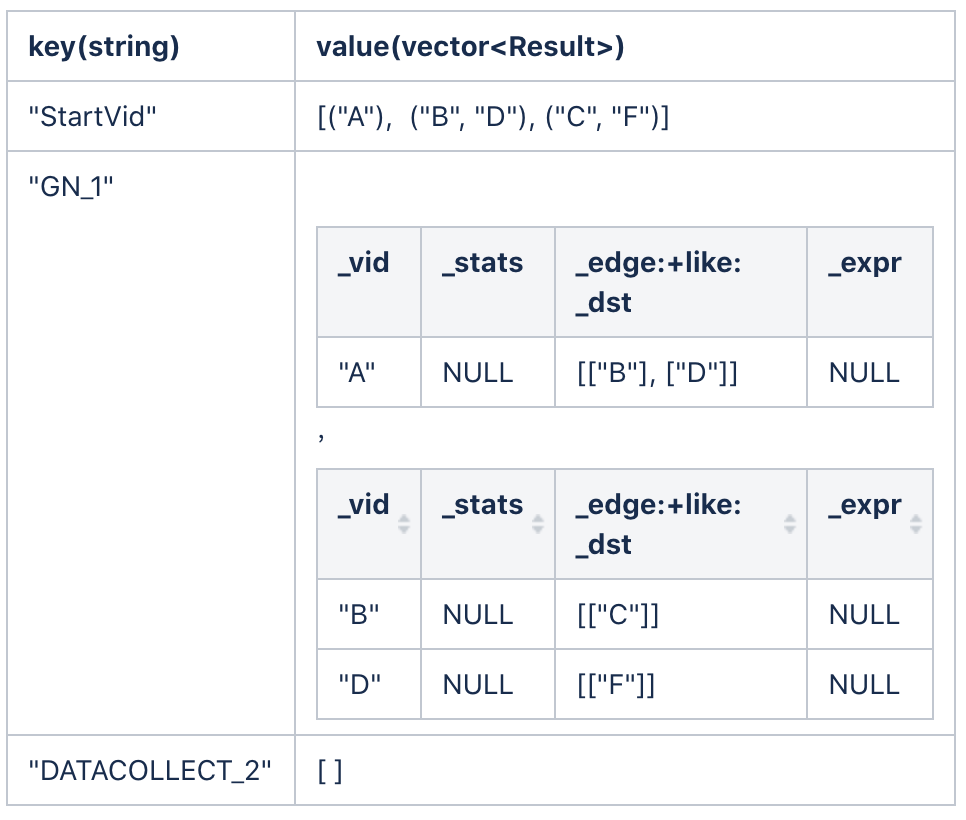After the third hop traversal, the ResultMap is as follows.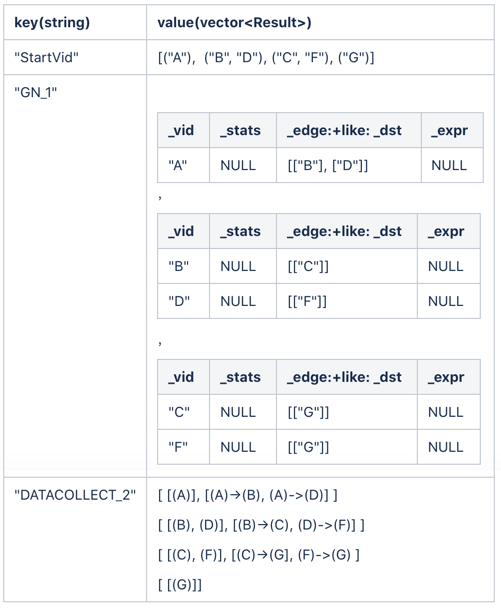Finally, `dataCollect` takes all the vertex sets and edge sets traversed in the process, assembles them into the final result, and returns it for users.

## Example

In this section, I will use an example to show the execution plan structure of a subgraph in NebulaGraph 2.0:

1. Open Nebula Console.
2. Switch to the `nba` graph space.
3. Run `EXPLAIN format="dot" GET SUBGRAPH 2 STEPS FROM 'Tim Duncan' IN like, serve`. Data of the DOT format is generated on Nebula Console.
4. Open the Graphviz Online website and copy and paste the DOT data on it.

The execution plan is shown as follows.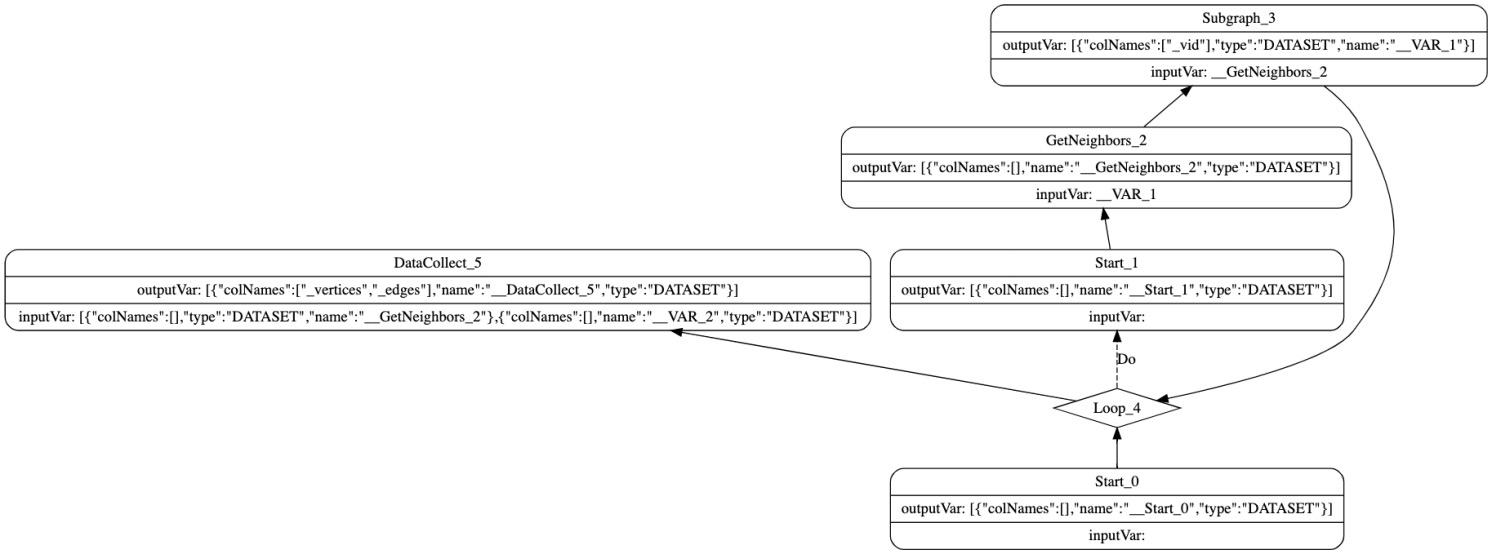In this plan, `depend` of `loop` depends on `Start_0`. However, because there is no multi-statement or PIPE, it is not necessary. ​ Welcome to join the NebulaGraph Slack channel to learn more about NebulaGraph.